Foxtable(狐表)用户栏目专家坐堂 → 会计凭证的设计和录入

共有851人关注过本帖树形打印复制链接

# 主题：会计凭证的设计和录入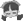21楼 | 信息 | 搜索 | 邮箱 | 主页 | UC加好友发短信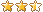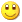Post By：2021/4/1 16:40:00 [只看该作者]

 一定要选定用哪套凭证的，有的软件在初启时选定，有的在设置凭证格式时选定，选定后另一套格式就不显了。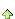22楼 | 信息 | 搜索 | 邮箱 | 主页 | UC加好友发短信Post By：2021/4/2 21:03:00 [只看该作者]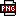此主题相关图片如下：7.png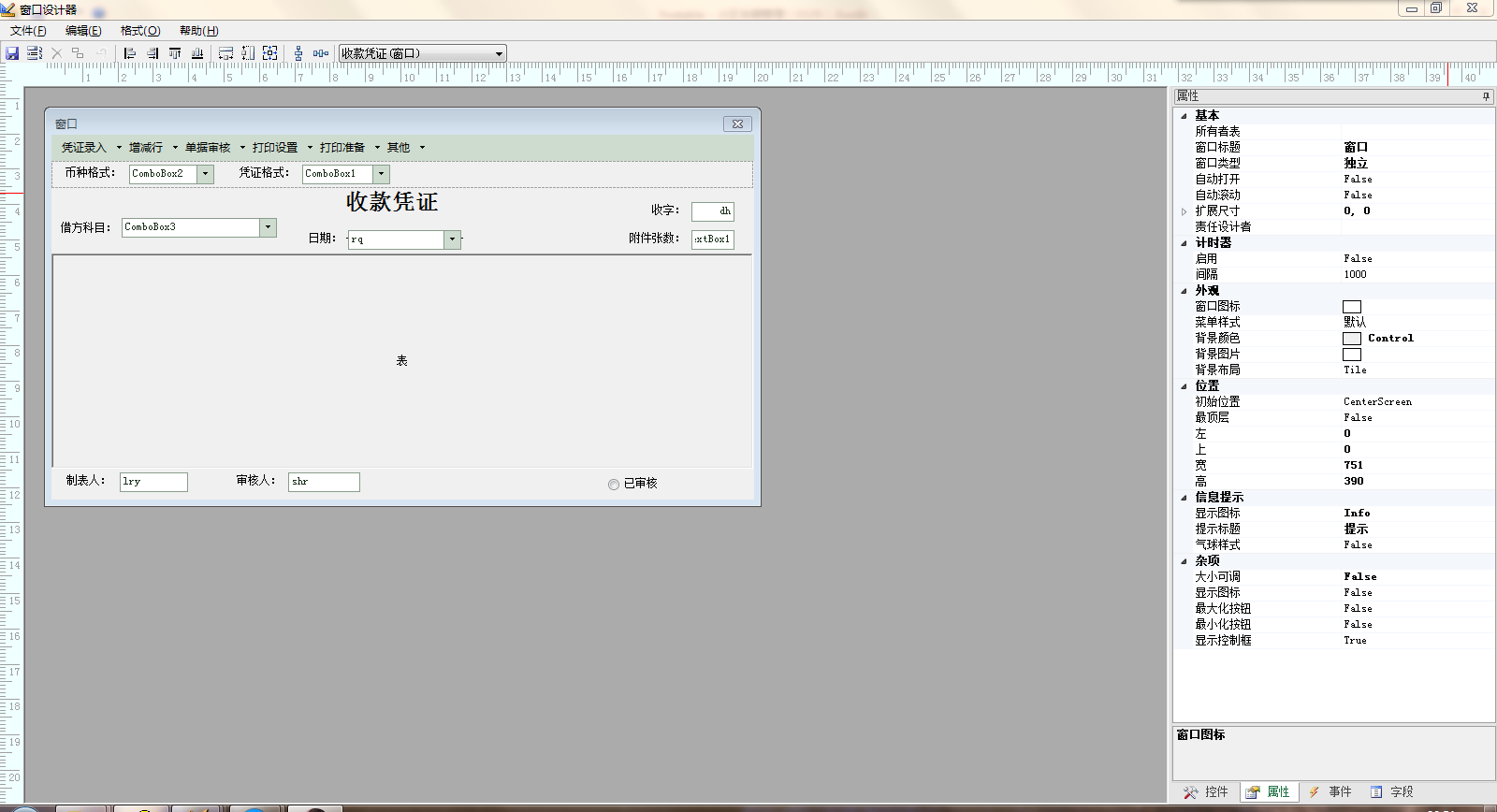此主题相关图片如下：3.png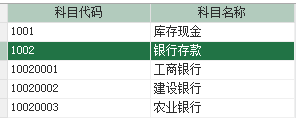我想把下图的现金、银行存款两个科目作为上图借方科目的下拉选项动态添加的数据源，如下显示：现金银行存款-工商行xx分行银行存款-建设银行xx分行银行存款-农业银行xx分行......请教怎样实现，看清楚是以会计科目表现金、银行存款科目为数据源的，只是只显示这两个科目。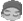23楼 | 信息 | 搜索 | 邮箱 | 主页 | UC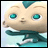加好友发短信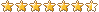Post By：2021/4/3 9:17:00 [只看该作者]

 借方科目控件enter事件dim lst as new List(of string)dim sr() as string = {"现金","银行存款"}dim sr2() as string = {"1001","1002"}for i as integer = 0 to sr.length - 1lst.add(sr(i))for each dr as datarow in datatables("表A").select("科目代码 like '" & sr2(i) & "%' and len(科目代码) =8")lst.add(sr(i) & "-" & dr("科目名称"))nextnexte.sender.ComboList = string.join("|",lst.toarray())24楼 | 信息 | 搜索 | 邮箱 | 主页 | UC加好友发短信Post By：2021/4/3 9:45:00 [只看该作者]

 下拉选项什么都没有显示。25楼 | 信息 | 搜索 | 邮箱 | 主页 | UC加好友发短信Post By：2021/4/3 9:46:00 [只看该作者]

 有了，放错了事件。26楼 | 信息 | 搜索 | 邮箱 | 主页 | UC加好友发短信Post By：2021/4/3 9:47:00 [只看该作者]

 还有，有时分行的名称太长了，控件显示能自动行高吗？27楼 | 信息 | 搜索 | 邮箱 | 主页 | UC加好友发短信Post By：2021/4/3 10:57:00 [只看该作者]

 不能的28楼 | 信息 | 搜索 | 邮箱 | 主页 | UC加好友发短信Post By：2021/4/6 10:54:00 [只看该作者]

 下载信息  [文件大小：   下载次数： ]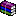点击浏览该文件:小区车辆管理（0406）.rar此主题相关图片如下：1.png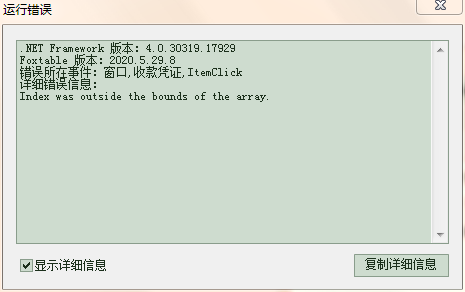'一.数据录入:
Select Case e.StripItem.Name
Case "保存" '保存代
If e.Form.Controls("rq").text = "" Then
messagebox.show("请选择日期")
Return
ElseIf Tables("收款凭证_Table1").Compute("Count([贷方科目])") = 0 Then
Return
Else
Dim r As DataRow = DataTables("收付转财务数据源").SQLFind("[字号] = '收' And [日期] = '" & e.Form.Controls("rq").text & "' And [凭证号] = '" & e.Form.Controls("dh").text & "'")
r = DataTables("收付转财务数据源").addnew
r("借或贷") = "借"
Dim Tel0 As String = e.Form.Controls("ComboBox3").text
Dim Parts0() As String = Tel0.Split("-")
r("借方科目") = Parts0(0)
r("二级科目") = Parts0(1)
r("三级科目") = Parts0(2)
r("四级科目") = Parts0(3)
r("五级科目") = Parts0(4)
r("借方金额") = Tables("收款凭证_Table1").Cols("贷方金额").GrandTotal
DataTables("收付转财务数据源").Save()
For Each dt As DataRow In DataTables("收款凭证_Table1").Select("[贷方科目] Is not null")
Dim Tel As String = dt("明细科目")
Dim Parts() As String = Tel.Split("-")
If e.Form.Controls("ComboBox2").text = e.form.controls("combobox2").items(0) And e.Form.Controls("ComboBox1").text = e.form.controls("combobox1").items(0) Then
dt("日期") = e.Form.Controls("rq").text
dt("凭证号") = e.Form.Controls("dh").text
dt("安日期生成单号辅助列") = e.Form.Controls("dh1").text
dt("附件张数") = e.Form.Controls("TextBox1").text
dt("录入员") = e.Form.Controls("lry").text
dt("字号") = "收"
dt("操作人姓名") = User.Name & " " & Date.Now
dt("二级科目") = Parts(0)
dt("三级科目") = Parts(1)
dt("四级科目") = Parts(2)
dt("五级科目") = Parts(3)
dt("借或贷") = "贷"
dt.Save
End If
Next
End If
End Select
我要把红色标注的借方增加到收付转财务数据源表中。
[此贴子已经被作者于2021/4/6 11:10:32编辑过]29楼 | 信息 | 搜索 | 邮箱 | 主页 | UC加好友发短信Post By：2021/4/6 10:59:00 [只看该作者]

 Dim Parts0() As String = Tel0.Split("-")    if   Parts0.length > 0 then     r("借方科目") = Parts0(0)       if   Parts0.length > 1 then      r("二级科目") = Parts0(1)30楼 | 信息 | 搜索 | 邮箱 | 主页 | UC加好友发短信Post By：2021/4/6 17:34:00 [只看该作者]此主题相关图片如下：2000.png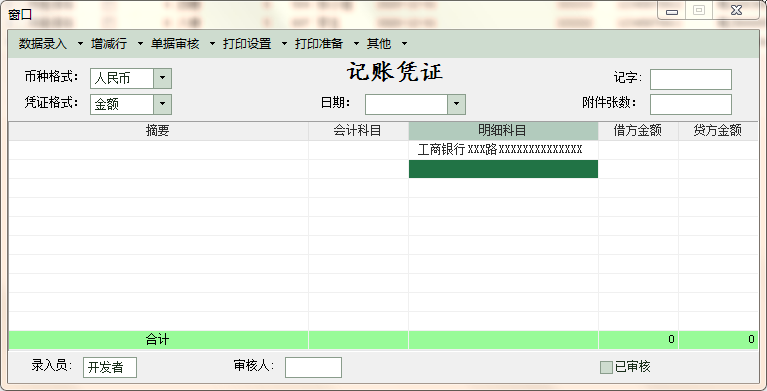不知什么原因，代码没变，原来没问题的，现在记账凭证窗口会计科目列没有了显示，收款凭证窗口没问题的，贷方科目能正常显示。Dim Vals() As String = e.Node.FullPath.Split("\")Dim tr As RowIf e.Form.DropTable IsNot Nothing Then '如果下拉窗口是从表中打开    tr = e.Form.DropTable.Current '获取此表的当前行Else '如果下拉窗口是通过DropdownBox打开    Dim nm As String = e.Form.DropDownBox.BindingField '获取绑定的字段    nm = nm.Split(".")(0) '获取绑定的表名    tr = Tables(nm).Current '获取此表的当前行End IfIf e.Form.DropTable IsNot Nothing Then    Select Case e.Form.DropTable.Name        Case "财务数据源","收付转财务数据源"            Select Case e.Node.Level                Case 0                    tr("会计科目") = Vals(0)                    tr("二级科目") = Nothing                    tr("三级科目") = Nothing                    tr("四级科目") = Nothing                    tr("五级科目") = Nothing                    e.Form.DropDownBox.Value = tr("会计科目")                    e.Form.DropDownBox.CloseDropdown()                Case 1                    tr("会计科目") = Vals(0)                    tr("二级科目") = Vals(1)                    tr("三级科目") = Nothing                    tr("四级科目") = Nothing                    tr("五级科目") = Nothing                    e.Form.DropDownBox.Value = tr("会计科目")                    e.Form.DropDownBox.CloseDropdown()                Case 2                    tr("会计科目") = Vals(0)                    tr("二级科目") = Vals(1)                    tr("三级科目") = Vals(2)                    tr("四级科目") = Nothing                    tr("五级科目") = Nothing                    e.Form.DropDownBox.Value = tr("会计科目")                    e.Form.DropDownBox.CloseDropdown()                Case 3                    tr("会计科目") = Vals(0)                    tr("二级科目") = Vals(1)                    tr("三级科目") = Vals(2)                    tr("四级科目") = Vals(3)                    tr("五级科目") = Nothing                    e.Form.DropDownBox.Value = tr("会计科目")                    e.Form.DropDownBox.CloseDropdown()                Case 4                    tr("会计科目") = Vals(0)                    tr("二级科目") = Vals(1)                    tr("三级科目") = Vals(2)                    tr("四级科目") = Vals(3)                    tr("五级科目") = Vals(4)                    e.Form.DropDownBox.Value = tr("会计科目")                    e.Form.DropDownBox.CloseDropdown()            End Select        Case "记账凭证_Table1","转账凭证_Table1"            Select Case e.Node.Level                Case 0                    tr("会计科目") = Vals(0)                    e.Form.DropDownBox.Value = tr("会计科目")                    e.Form.DropDownBox.CloseDropdown()                Case 1                    tr("会计科目") = Vals(0)                    tr("明细科目") = Vals(1).SubString(8,Vals(1).Length - 8)                    e.Form.DropDownBox.Value = tr("会计科目")                    e.Form.DropDownBox.CloseDropdown()                Case 2                    tr("会计科目") = Vals(0)                    tr("明细科目") = Vals(1).SubString(8,Vals(1).Length - 8) & "-" & Vals(2).SubString(12,Vals(2).Length - 12)                    e.Form.DropDownBox.Value = tr("会计科目")                    e.Form.DropDownBox.CloseDropdown()                Case 3                    tr("会计科目") = Vals(0)                    tr("明细科目") = Vals(1).SubString(8,Vals(1).Length - 8) & "-" & Vals(2).SubString(12,Vals(2).Length - 12) & "-" & Vals(3).SubString(16,Vals(3).Length - 16)                    e.Form.DropDownBox.Value = tr("会计科目")                    e.Form.DropDownBox.CloseDropdown()                Case 4                    tr("会计科目") = Vals(0)                    tr("明细科目") = Vals(1).SubString(8,Vals(1).Length - 8) & "-" & Vals(2).SubString(12,Vals(2).Length - 12) & "-" & Vals(3).SubString(16,Vals(3).Length - 16) & "-" & Vals(4).SubString(20,Vals(4).Length - 20)                    e.Form.DropDownBox.Value = tr("会计科目")                    e.Form.DropDownBox.CloseDropdown()            End Select [此贴子已经被作者于2021/4/6 17:36:06编辑过]总数 68 上一页 1 2 3 4 5 6 7 下一页